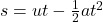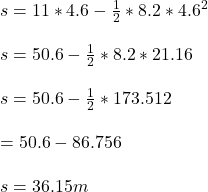## The driver is looking at her phone and has a total reaction time of 4.6 seconds as the car is moving at a constant speed of 11m/s. If the d

Question

The driver is looking at her phone and has a total reaction time of 4.6 seconds as the car is moving at a constant speed of 11m/s. If the driver slams in her brake and slow down at a rate of -8.2m/s, what is the stopping distance for a car in this situation?

in progress 0
7 months 2021-07-20T21:44:45+00:00 1 Answers 18 views 0

## The stopping distance is 36.15m

Explanation:

In this exercise we are going to apply the second equation of motion to model the situationGiven data

time in seconds= 4.6 seconds

speed v= 11 m/s

deacceleration a= 8.2m/s

Substituting this values into the expression for distance we have